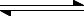Chemguide: Core Chemistry 14 - 16 ``` ``` The effect of changing the pressure in a dynamic equilibrium involving gases This page looks at what happens when you change the pressure in a dynamic equilibrium involving gases. It only applies where gases are involved. It assumes that you have already read the page about introducing reversible reactions and equilibria - in particular the last part of that page about Le Chatelier's Principle. ``` ``` Pressure in gases Pressure is caused by gas molecules hitting the walls of the container. If you have a gas at a fixed temperature and then increase the number of molecules in a given volume, the pressure will increase because there are more collisions with the walls of the container. And, of course, if you decrease the number of molecules, the pressure will fall because the number of collisions with the wall will fall. ``` ``` The effect of pressure on the equilibrium in the production of ammonia N2(g) + 3H2(g)2NH3(g) What would happen if you increased the pressure on this equilibrium? According to Le Chatelier, the position of equilibrium would move to counter the change. So how does the position shift in this case? The forward reaction produces two molecules of ammonia from a total of four molecules of nitrogen and hydrogen. There is a fall in the total number of molecules hitting the walls in the reaction vessel. So a shift in the equilibrium to the right will tend to reduce the pressure. In this reaction, increasing the pressure on the system will cause the equilibrium to move towards the formation of ammonia. The reaction is actually done at very high pressures - of the order of 200 atmospheres. It isn't really relevant in this case, because you want to form ammonia, but for completeness, let's think about what the effect of lowering the pressure would be. If you lowered the pressure, Le Chatelier suggests that the equilibrium would shift to try to counter that. It can do that by producing more molecules of gas. In this case, the position of equilibrium would shift to the left. How do you phrase this if you are avoiding the words Le Chatelier? Increasing the pressure causes the position of equilibrium to move towards the side with the fewer gas molecules. Decreasing the pressure causes the position of equilibrium to move towards the side with the greater number of gas molecules. ``` ``` The effect of pressure on the equilibrium in the production of sulfuric acid 2SO2(g) + O2(g)2SO3(g) What sort of pressure would you expect to use to maximise the amount of sulfur trioxide formed? There are 3 molecules on the left-hand side but only 2 on the right. If you increased the pressure, the position of equilibrium will move towards the side with the fewer molecules - and that's exactly what you want. You would therefore expect this to be done at a high pressure. But it isn't! It is actually done at a pressure between 1 and 2 atmospheres. Even at ordinary pressures, there is a 99.5% conversion of sulfur dioxide into sulfur trioxide. High pressures are expensive to produce. You need very thick walled pipes and reaction vessels, and use a lot of energy to compress the gases. In this particular case, it isn't worth it just to get a tiny extra percentage conversion. We will look at this and the ammonia case again and in even more detail in the section on Large Scale Chemistry. ``` ``` The effect of pressure on the hydrogen iodide / hydrogen and iodine equilibrium If hydrogen iodide gas is heated it produces this equilibrium: 2HI(g)H2(g) + I2(g) What would happen to the position of equilibrium if you changed the pressure this time? If you look at the equation, there are the same number of molecules on both sides of the equilibrium. If you increased the pressure, there is no movement of the position of the equilibrium that could help to reduce it again. If there are the same number of molecules of gas on both sides of the equation, changing the pressure makes no difference to the position of equilibrium. ``` ``` Where would you like to go now? To the reversible reactions and equilbria menu . . . To the Chemistry 14-16 menu . . . To Chemguide Main Menu . . . ``` ``` © Jim Clark 2020